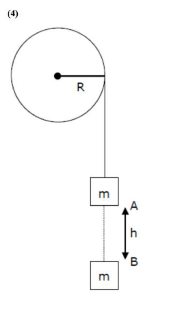# A cord is wound round the circumference of wheel of radius r.Question:

A cord is wound round the circumference of wheel of radius $\mathbf{r}$. The axis of the wheel is horizontal and the moment of inertia about it is I. A weight $\mathrm{mg}$ is attached to the cord at the end. The weight falls from rest. After falling through a distance 'h', the square of angular velocity of wheel will be:

1. $\frac{2 g h}{I+m r^{2}}$

2. 2gh

3. $\frac{2 m g h}{I+2 m r^{2}}$

4. $\frac{2 m g h}{I+m r^{2}}$

Correct Option: , 4

Solution:using energy conservation between $\mathrm{A}$ and $\mathrm{B}$ point

$m g h=\frac{1}{2} m(w R)^{2}+\frac{1}{2} I \omega^{2}$

$2 \mathrm{mgh}=\left(\mathrm{MR}^{2}+\mathrm{I}\right) \omega^{2}$

$\omega^{2}=\frac{2 m g h}{I+M R^{2}}$